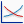# Sensitivity Plot

If you have performed a Sensitivity Analysis with Slide2, then the results are viewed with the Sensitivity Plot option in the Slide2 Interpret program.

To create a Sensitivity Plot:

1. Select Sensitivity Plotfrom the toolbar or the Statistics menu.
2. In the Sensitivity Plot dialog:
• Select the Analysis Method for which you would like to view results.
• Use the checkboxes in the Data to Plot area, to select the Sensitivity Analysis variables that you would like to plot.
3. Select the Plot button to generate the Sensitivity Plot.
4. Once a plot is generated, there are several options available, if you right-click the mouse on the plot. See below for details.

## Sensitivity Plot

A Sensitivity Plot is simply a plot of safety factor against the percentage change in specified model parameters. On sensitivity plots, the gradient of a curve for a parameter indicates the effect that parameter has on the factor of safety. Steeper rising or falling curves indicate greater influence on the factor of safety. A relatively "flat" curve indicates that a variable has little or no effect on the factor of safety.

## Sensitivity Plot of two variables### Notes:

• Remember that each curve on a Sensitivity Plot is obtained by varying ONLY ONE PARAMETER while keeping all other variables constant (at their mean value).
• Each curve is generated by varying the parameter between the user-specified minimum and maximum values, in 50 equal increments, and calculating the safety factor for the Deterministic Global Minimum slip surface, at each value of the variable.
• The horizontal axis of a Sensitivity Plot is expressed in terms of the Percent of Range of the variable. This is the relative difference between the Minimum value of a variable (0 percent) and the Maximum value of a variable (100 percent).
• If you are only plotting a single variable on a Sensitivity Plot, then the horizontal axis will be in terms of the actual value of the variable, rather than the Percent of Range.
• All curves on a Sensitivity Plot will always intersect at Percent of Range = 50%. This always corresponds to the MEAN value of ALL variables.
• If the minimum and maximum values of each variable are an equal distance from the MEAN value, then each curve on a Sensitivity Plot will cover the full range of 0 to 100%.
• If the minimum and maximum values of a variable are NOT an equal distance from the MEAN, then the curve on the Sensitivity Plot will not span the full range of 0 to 100% (this is done to ensure that the Percent of Range = 50%, ALWAYS represents the MEAN value of all variables, even if the minimum and maximum are not equal distances from the MEAN.)

## Right-Click Options

Several options are available if you right-click on a Sensitivity Plot. For example:

• Select the Change Plot Data option to change the data viewed on the plot.
• Select the Chart Properties option to customize the appearance of the plot.
• The Sampler option allows you to graphically determine the coordinates of any points on the Sensitivity Plot curves. For more information, see the discussion of the Sampler for the Cumulative Plot, as the Sensitivity Plot Sampler operates in a similar manner.

Experiment with the different options available in the right-click menu.